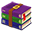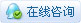221浏览

# Mind+Python turtle 课程——06 小海龟走迷宫实现功能 知识点 任务一：添加迷宫背景及起止点 turtle库基础函数使用 任务二：添加鼠标控制功能 布尔类型、条件判断、鼠标控制函数的使用、逻辑运算符的使用

1、任务分析

2、编写程序

 from turtle import *#导入turtle库中所有工具#绘制背景------------------------------------------screen = Screen()#赋值screen对象width,height = 800,720#设定宽度和高度screen.setup(width,height)#以设定好的宽度和高度来创建屏幕screen.title("小海龟走迷宫")#设定屏幕标题screen.bgcolor("white")#设定屏幕背景色screen.bgpic('迷宫4.gif')#添加迷宫背景图screen.delay(0)#这里要设延时为0，否则很卡#绘制海龟------------------------------------------t = Turtle('turtle')#创建一个海龟t.shapesize(0.8)#设定海龟尺寸大小t.speed(0)#设定海龟速度为最大t.color('blue')#设定海龟颜色为蓝色t.penup()#抬笔t.goto(0,0)#使海龟移动到指定位置作为起点t.write("起点",font=('微软雅黑', 14, 'normal'))#设定海龟所在为起点#绘制终点------------------------------------------yuan = Turtle('circle')#创建一个圆作为终点标志yuan.shapesize(0.7)#设定圆的尺寸大小[size=12.0000pt]，默认值的0.7倍yuan.color('red')#设定圆的颜色为红色yuan.penup()#抬笔yuan.goto(-300,120)#使圆移动到指定位置[size=12.0000pt]，作为迷宫的终点yuan.write("终点",font=('微软雅黑', 14, 'normal'))#添加终点标记done()#用来停止画笔绘制，但绘图窗体不关闭
3、运行效果4、代码释义
4.1turtle库中各类Turtle()函数的使用

 #绘制海龟------------------------------------------t = Turtle('turtle')#创建一个海龟t.shapesize(0.8)#设定海龟尺寸大小t.speed(0)#设定海龟速度为最大t.color('blue')#设定海龟颜色为蓝色t.write("起点",font=('微软雅黑',[size=12.0000pt] 14,[size=12.0000pt] 'normal'))#设定海龟所在为起点#绘制终点------------------------------------------yuan = Turtle('circle')#创建一个圆作为终点标志yuan.shapesize(0.7)#设定圆的尺寸大小yuan.color('red')#设定圆的颜色为红色yuan.penup()#抬笔yuan.goto(-300,120)#使圆移动到指定位置yuan.write("终点",font=('微软雅黑', 14, 'normal'))#添加终点标记

4.1.1代码解释

①　使用shapesize()命令设定海龟和圆的尺寸大小
②　使用color()命令设定颜色
③　使用speed()命令设定移动速度
④　使用penup()命令设定抬笔
⑤　使用goto()命令来移动到指定位置
⑥　最后使用write()命令来书写的文字。

4.1.2使用方法

 t = Turtle('turtle')#创建一个海龟yuan = Turtle('circle')#创建一个圆作为终点标志

4.1.3注意事项

 t.shapesize(0.8)#设定海龟尺寸大小yuan.shapesize(0.7)#设定圆的尺寸大小

 t.color('blue')#设定海龟颜色为蓝色yuan.color('red')#设定圆的颜色为红色

 t.write("起点",font=('微软雅黑',[size=12.0000pt] 14,[size=12.0000pt] 'normal'))#设定海龟所在为起点yuan.write("终点",font=('微软雅黑', 14, 'normal'))#添加终点标记

1、任务分析bx=mx-rx-2
by=my-rx-2X=bx - screen.window_width() //2
Y=screen.window_height() //2 - by
2、编写程序

 from turtle import *#导入turtle库中所有工具#绘制背景------------------------------------------screen = Screen()#赋值screen对象width,height = 800,720#设定宽度和高度screen.setup(width,height)#以设定好的宽度和高度来创建屏幕screen.title("小海龟走迷宫")#设定屏幕标题screen.bgcolor("white")#设定屏幕背景色screen.bgpic('迷宫4.gif')#添加迷宫背景图screen.delay(0)#这里要设延时为0，否则很卡#绘制起点------------------------------------------t = Turtle('turtle')#创建一个海龟t.shapesize(0.8)#设定海龟尺寸大小t.speed(0)#设定海龟速度为最大t.color('blue')#设定海龟颜色为蓝色t.penup()#抬笔t.goto(0,0)#使海龟移动到指定位置作为起点t.write("起点",font=('微软雅黑', 14, 'normal'))#设定海龟所在为起点#绘制终点------------------------------------------yuan = Turtle('circle')#创建一个圆作为终点标志yuan.shapesize(0.7)#设定圆的尺寸大小yuan.color('red')#设定圆的颜色为红色yuan.penup()#抬笔yuan.goto(-300,120)#使圆移动到指定位置[size=12.0000pt]，作为迷宫的终点yuan.write("终点",font=('微软雅黑', 14, 'normal'))#添加终点标记#    """获取鼠标指针的坐标"""root = screen._root#获取根窗口对象cv = screen._canvas#获取画布对象while True:#永久循环    mx = root.winfo_pointerx()#鼠标指针相对于计算机屏幕的x坐标    my = root.winfo_pointery()#鼠标指针相对于计算机屏幕的y坐标[size=12.0000pt]     rx = cv.winfo_rootx()#画布到计算机屏幕最左边距离    ry = cv.winfo_rooty()#画布到计算机屏幕最上边距离     bx = mx - rx - 2#画布边框宽度是2，所以要减去2    by = my - ry - 2#画布边框高度是2，所以要减去2     x = bx - screen.window_width() //2#转换成在海龟画图坐标系中x坐标    y = screen.window_height() //2 - by#转换成在海龟画图坐标系中y坐标[size=12.0000pt]     if x>=-300 and x<=-290 and y<=120 and y>=110:#如果海龟到了终点侧        yuan.write("      到啦",font=('微软雅黑', 14, 'normal'))#提示到达        done()#用来停止画笔绘制，但绘图窗体不关闭    else:#否则        t.goto(x,y)#使海龟移动到指定坐标        #screen.update()#刷新屏幕显示    if x>=0 and x<=10 and y>=0 and y<=10:#如果海龟到了起点        t.pendown()        t.write("      可以开始啦",font=('微软雅黑', 14, 'normal'))#提示到达
3、运行效果4、代码释义
4.1代码中布尔类型的使用

 while[size=12.0000pt] True:#永久循环

4.1.1代码释义

4.1.2什么是布尔类型

4.1.3什么是基本数据类型
python中，我们编写的代码程序都是数据，为了便于计算机识别，我们将存储在python中的数据划分为多种类型。例如，常用的数字类型有“13”，“250”。布尔类型“true”，“false”。字符串类型“aaaa”，“hello world”等。

4.2代码中键盘函数的使用

 [size=12.0000pt]root[size=12.0000pt] [size=12.0000pt]=[size=12.0000pt] screen._root#获取窗口对象cv[size=12.0000pt] [size=12.0000pt]=[size=12.0000pt] screen._canvas#获取画布对象while[size=12.0000pt] True:#永久循环    m[size=12.0000pt]x[size=12.0000pt] [size=12.0000pt]=[size=12.0000pt] root.winfo_pointerx()#鼠标指针相对于计算机屏幕的x坐标    m[size=12.0000pt]y[size=12.0000pt] [size=12.0000pt]=[size=12.0000pt] root.winfo_pointery()#鼠标指针相对于计算机屏幕的y坐标    rx[size=12.0000pt] [size=12.0000pt]=[size=12.0000pt] cv.winfo_rootx()#画布到计算机屏幕最左边距离    ry[size=12.0000pt] [size=12.0000pt]=[size=12.0000pt] cv.winfo_rooty()#画布到计算机屏幕最上边距离    b[size=12.0000pt]x[size=12.0000pt] [size=12.0000pt]= m[size=12.0000pt]x[size=12.0000pt] [size=12.0000pt]-[size=12.0000pt] rx[size=12.0000pt] [size=12.0000pt]-[size=12.0000pt] [size=12.0000pt]2#画布边框宽度是2，所以要减去2    b[size=12.0000pt]y[size=12.0000pt] [size=12.0000pt]= m[size=12.0000pt]y[size=12.0000pt] [size=12.0000pt]-[size=12.0000pt] ry[size=12.0000pt] [size=12.0000pt]-[size=12.0000pt] [size=12.0000pt]2#画布边框高度是2，所以要减去2    [size=12.0000pt]x[size=12.0000pt] [size=12.0000pt]= b[size=12.0000pt]x[size=12.0000pt] [size=12.0000pt]-[size=12.0000pt] screen.window_width()[size=12.0000pt] //2#转换成在海龟画图坐标系中x坐标    [size=12.0000pt]y[size=12.0000pt] [size=12.0000pt]=[size=12.0000pt] screen.window_height()[size=12.0000pt] //2[size=12.0000pt] [size=12.0000pt]- b[size=12.0000pt]y#转换成在海龟画图坐标系中y坐标

4.3代码中if...else语句的使用

 if x>=-300 and x<=-290 and y<=120 and y>=110:#如果海龟到了终点侧        yuan.write("      到啦",font=('微软雅黑', 14, 'normal'))#提示到达        done()#用来停止画笔绘制，但绘图窗体不关闭else:#否则        t.goto(x,y)#使海龟移动到指定坐标if x>=0 and x<=5 and y>=0 and y<=5:#如果海龟到了起点        t.pendown()#落笔        t.write("      可以开始啦",font=('微软雅黑', 14, 'normal'))#提示到达

4.3.1代码解释

4.3.2if与if...else条件判断语句的区别
if语句与if...else语句都是条件判断语句，在上节课我们已经了解了if语句的使用，在需要进行判断的情况只有一个时，我们使用if语句，而当遇到只能二选一的条件时，我们就要使用if...else语句来实现了。这里if表示如果，else表示否则。我们还是举几个生活中常见的例子：

1. 如果闹钟响了，我们就起床；否则，我们继续睡觉。
2. 如果下课铃声响了，我们就下课；否则，我们继续上课。
3. 如果输入的用户名和密码正确，提示登录成功；否则，提示密码错误。

4.3.3if...else语句使用方法
 if 条件表达式：    语句块else:    语句块if...else 语句的执行流程图

4.3.4 and逻辑运算符

 if x>=-300 and x<=-290 and y<=120 and y>=110:#如果海龟到了终点侧......if x>=0 and x<=5 and y>=0 and y<=5:#如果海龟到了起点......

a and b
 And（逻辑与）

A or b
Or(逻辑或)

！a
Not（逻辑非）

 from[size=12.0000pt] turtle[size=12.0000pt] import[size=12.0000pt] [size=12.0000pt]*#导入turtle库中所有工具#绘制背景------------------------------------------[size=12.0000pt]screen[size=12.0000pt] [size=12.0000pt]=[size=12.0000pt] Screen()#赋值screen对象width,height[size=12.0000pt] [size=12.0000pt]=[size=12.0000pt] 800,720#设定宽度和高度screen.setup(width,height)#以设定好的宽度和高度来创建屏幕screen.title("小海龟走迷宫")#设定屏幕标题screen.bgcolor("white")#设定屏幕背景色screen.bgpic('迷宫4.gif')#添加迷宫背景图screen.delay(0)#这里要设延时为0，否则很卡#绘制起点------------------------------------------[size=12.0000pt]t[size=12.0000pt] [size=12.0000pt]=[size=12.0000pt] Turtle('turtle')#创建一个海龟t.shapesize(0.8)#设定海龟的尺寸大小t.speed(0)#设定海龟速度为最大t.color('black')#设定海龟颜色为黑t.write("起点",font=('微软雅黑', 14, 'normal'))#设定海龟所在为起点#绘制终点------------------------------------------yuan[size=12.0000pt] [size=12.0000pt]=[size=12.0000pt] Turtle('circle')#创建一个圆作为终点yuan.shapesize(0.7)#设定圆的尺寸大小yuan.color('red')#设定圆的颜色为红色yuan.penup()#抬笔yuan.goto(-300,120)#使圆移动到指定位置yuan.write("终点",font=('微软雅黑',[size=12.0000pt] 14,[size=12.0000pt] 'normal'))#添加终点标记screen.onclick(t.goto)#使海龟移动到鼠标左键单机处done()#用来停止画笔绘制，但绘图窗体不关闭6、小海龟走迷宫.rar (2.59 MB, 下载次数: 10)### 追梦的少年中级技师

 6666666666

 创意十足

 您需要登录后才可以回帖 登录 | 立即注册 本版积分规则 回帖并转播 回帖后跳转到最后一页

[[wsData.name]]

#### 硬件清单

• [[d.name]]#### 楼主的其它帖子